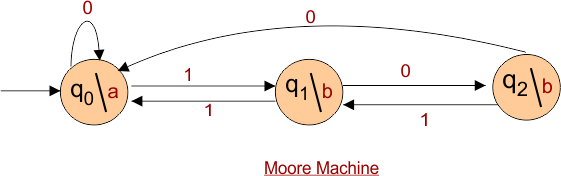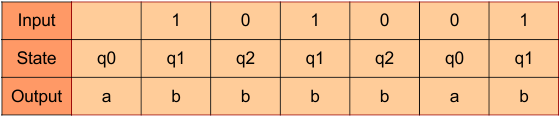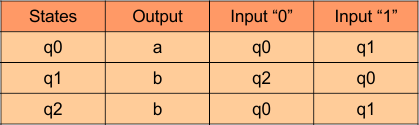Select Page

# Automata Moore Machine

As we already covered the topics of DFA and NFA. Both DFA and NFA are the automata machines which take the input and do not give the output. Both DFA and NFA just check the acceptance of the given string. But the Automata Moore Machine and Mealy take the input and give the output also. There is no final state in Moore Machine.

Condition: For Moore machine, there must be a dedicated path for each input from each state like DFA.

Moore and Mealy machines takes an input string (i.e. 11001) and given output (i.e. aabbab).

• Each state of Moore or mealy machine gives an output. Output is denoted by Output symbol.
• Initial state always gives an output. Remaining outputs depends on transition function.
 Important: Output of Moore machine is always one greater than input. If the input length is three (i.e. 011) then output length will be four (i.e. aabb). Because initial state always gives an output without an input.

## Formal Definition of Moore Machine

Moore and mealy machines accept all regular languages. The output depends only on the current state of the machine. The output is represented by current state separated by “/”.

Moore machine can be described by 6 tuples (Q, q0, ∑, O, δ, λ) where,

• Q: finite set of states
• q0: initial state of machine
• ∑: finite set of input symbols
• O: output alphabet
• δ: transition function where Q × ∑ → Q
• λ: output function where Q → O

## Example of Moore Machine

Consider the following Moore Machine### Explanation of Moore Machine Tuples

Explanation of 6 tuples (Q, q0, ∑, δ, O, λ) is given below

1. q0, q1, q2 are the states of given Moore machine.

2. q0 is the initial state

3. 0,1 are the inputs (∑) values for transition.

4. Transition function is performed on input and gives a state move.

5. O is the output, where a, b are output symbols which are separated by “/” from state symbol.

6. Output function (λ) gives the output. As in above Moore Machine

• Initial state q0 gives output “a”
• If at state q0 and input “1” is given then state change to q1 and q1 will give the “b” output.
• In the same way, If at state q1 and input “1” is given then state change to q2 and q2 will give the “b” output.
• Similarly, if at state q2 and input “0” is given then state change to q0 and q0 will give the “a” output. And so on…

### Input to Output in Moore Machine

For instance if the input “101001” is given to above Moore machine then output will be “abbbbab”. First “a” of output comes without input. That’s why output is always greater than input length by 1. And remaining output comes after consuming each input. As shown in the following table.### Transition table of Moore Machine

As q0 gives  output “a”, q1 gives  output “b”, and q2 gives  output “b”. Transition for each input is also covered in DFA and NFA table. So, Transition table for given Moore Machine is given belowHelp Other’s By Sharing…